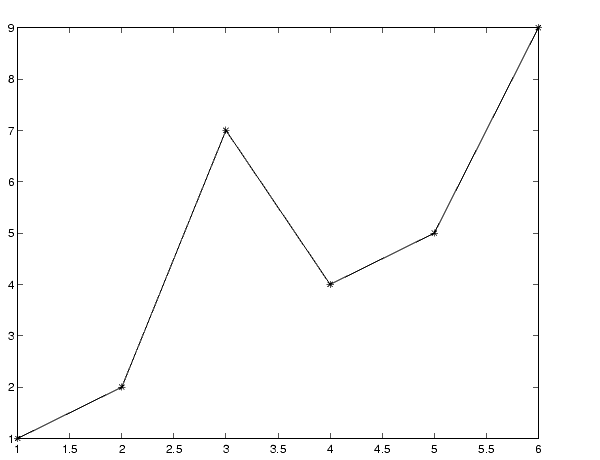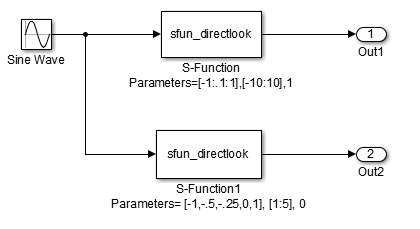## Write Fully Inlined S-Functions with mdlRTW Routine

You can inline more complex S-functions by using the S-function `mdlRTW` routine. The `mdlRTW` routine provides the code generation process with more information about how the S-function is to be inlined by creating a parameter record of a non-tunable parameter for use with a TLC file. The `mdlRTW` routine places information in the `model.rtw` file. The `mdlRTW` function is described in the text file `matlabroot/simulink/src/sfuntmpl_doc.c`.

To use the `mdlRTW` function, take steps to create a direct-index lookup S-function. Lookup tables are collections of ordered data points of a function. Typically, these tables use some interpolation scheme to approximate values of the associated function between known data points. To incorporate the example lookup table algorithm into a Simulink® model, the first step is to write an S-function that executes the algorithm in `mdlOutputs`. To produce the most efficient code, the next step is to create a corresponding TLC file to eliminate computational overhead and improve the speed of the lookup computations.

The Simulink product provides support for general-purpose lookup 1-D, 2-D, and n-D algorithms. You can use these algorithms as they are or create a custom lookup table S-function to fit your requirements. You can create a 1-D lookup S-function, `sfun_directlook.c`, and its corresponding inlined `sfun_directlook.tlc` file (see Target Language Compiler for more details). You can:

• Error check of S-function parameters.

• Cache information for the S-function that does not change during model execution.

• Use the `mdlRTW` function to customize the code generator to produce the optimal code for a given set of block parameters.

• Create a `TLC` file for an S-function that either fully inlines the lookup table code or calls a wrapper function for the lookup table algorithm.

### S-Function RTWdata

`RTWdata` is a property of blocks, which can be used by the Target Language Compiler when inlining an S-function. `RTWdata` is a structure of character vectors that you can attach to a block. `RTWdata` is saved with the model and placed in the `model.rtw` file when you generate code. For example, this set of MATLAB® commands:

```mydata.field1 = 'information for field1'; mydata.field2 = 'information for field2'; set_param(gcb,'RTWdata',mydata) get_param(gcb,'RTWdata') ```

produces this result:

```ans = field1: 'information for field1' field2: 'information for field2'```

The information for the associated S-Function block inside the `model.rtw` file is:

```Block {   Type "S-Function"   RTWdata {     field1 "information for field1"     field2 "information for field2"   }```

Note

`RTWdata` is saved in the model file for S-functions that are not linked to a library. However, `RTWdata` is not persistent for S-Function blocks that are linked to a library.

### Direct-Index Lookup Table Algorithm

The 1-D lookup table block provided in the Simulink library uses interpolation or extrapolation when computing outputs. In this example, you create a lookup table that directly indexes the output vector (y-data vector) based on the current input (x-data) point.

This direct 1-D lookup example computes an approximate solution p(x)to a partially known function f(x) at x=x0, given data point pairs (x,y) in the form of an x-data vector and a y-data vector. For a given data pair (for example, the i'th pair), y_i = f(x_i). It is assumed that the x-data values are monotonically increasing. If x0 is outside the range of the x-data vector, the first or last point is returned.

The parameters to the S-function are:

`XData, YData, XEvenlySpaced`

`XData` and `YData` are double vectors of equal length representing the values of the unknown function. `XDataEvenlySpaced` is a scalar, `0.0` for false and `1.0` for true. If the `XData` vector is evenly spaced, `XDataEvenlySpaced` is `1.0` and more efficient code is generated.

The graph shows how the parameters `XData=[1:6]`and `YData=[1,2,7,4,5,9]` are handled. For example, if the input (x-value) to the S-Function block is 3, the output (y-value) is 7.### Direct-Index Lookup Table Example

Improve the lookup table by inlining a direct-index S-function with a TLC file. This direct-index lookup table S-function does not require a TLC file. The example uses a TLC file for the direct-index lookup table S-function to reduce the code size and increase efficiency of the generated code.

Implementation of the direct-index algorithm with an inlined TLC file requires the S-function main module, `sfun_directlook.c` and a corresponding `lookup_index.c` module. The `lookup_index.c` module contains the `GetDirectLookupIndex` function that is used to locate the index in the `XData` for the current `x` input value when the `XData` is unevenly spaced. The `GetDirectLookupIndex` routine is called from the S-function and the generated code. The example uses the wrapper concept for sharing C/C++ code between Simulink MEX-files and the generated code.

If the `XData` is evenly spaced, then both the S-function main module and the generated code contain the lookup algorithm to compute the y-value of a given x-value because the algorithm is short.

The inlined TLC file is `sfun_directlook.tlc`, which is used to either perform a wrapper call or embed the optimal C/C++ code for the S-function. (See the example in mdlRTW Usage).

### Error Handling

In `sfun_directlook.tlc`, the `mdlCheckParameters` routine verifies that:

• The new parameter settings are valid.

• `XData` and `YData` are vectors of the same length containing real, finite numbers.

• `XDataEvenlySpaced` is a scalar.

• The `XData` vector is a monotonically increasing vector and evenly spaced.

The `mdlInitializeSizes` function explicitly calls `mdlCheckParameters` after it verifies the number of parameters passed to the S-function. After the Simulink engine calls `mdlInitializeSizes`, it then calls `mdlCheckParameters` whenever you change the parameters or reevaluate them.

### User Data Caching

In `sfun_directlook.tlc`, the `mdlStart` routine shows how to cache information that does not change during the simulation or while the generated code is executing. The example caches the value of the `XDataEvenlySpaced` parameter in `UserData`, a field of the `SimStruct`. The following line in `mdlInitializeSizes` instructs the Simulink engine to disallow changes to `XDataEvenlySpaced`.

`ssSetSFcnParamTunable(S, iParam, SS_PRM_NOT_TUNABLE);`

During execution, `mdlOutputs` accesses the value of `XDataEvenlySpaced` from `UserData` rather than calling the `mxGetPr` MATLAB API function.

### mdlRTW Usage

The code generator calls the `mdlRTW` routine while generating the `model.rtw` file. To produce optimal code for your Simulink model, you can add information to the `model.rtw` file about the mode in which your S-Function block is operating.

The example adds parameter settings to the `model.rtw` file. The parameter settings do not change during execution. In this case, the `XDataEvenlySpaced` S-function parameter cannot change during execution (`ssSetSFcnParamTunable` was specified as false (`0`) for it in `mdlInitializeSizes`). The parameter setting (`XSpacing`) uses the function `ssWriteRTWParamSettings`.

Because `xData` and `yData` are registered as run-time parameters in `mdlSetWorkWidths`, the code generator writes to the `model.rtw` file automatically.

Before examining the S-function and the inlined TLC file, consider the generated code for this model.The model uses evenly spaced `XData` in the top S-Function block and unevenly spaced `XData` in the bottom S-Function block. When creating this model, specify the following commands for each S-Function block.

```set_param('sfun_directlook_ex/S-Function','SFunctionModules','lookup_index') set_param('sfun_directlook_ex/S-Function1','SFunctionModules','lookup_index')```

The build process uses the module `lookup_index.c` when creating the executable.

When generating code for this model, the code generator uses the S-function `mdlRTW` method to generate a `model.rtw` file with the value `EvenlySpaced` for the `XSpacing` parameter for the top S-Function block and the value `UnEvenlySpaced` for the `XSpacing` parameter for the bottom S-Function block. The TLC-file uses the value of `XSpacing` to determine what algorithm to include in the generated code. The generated code contains the lookup algorithm when the `XData` is evenly spaced, but calls the `GetDirectLookupIndex` routine when the `XData` is unevenly spaced. The generated `model.c` or `model.cpp` code for the lookup table example model is similar to this code:

```/* * sfun_directlook_ex.c * * Code generation for Simulink model * "sfun_directlook_ex.slx". * ... */ #include "sfun_directlook_ex.h" #include "sfun_directlook_ex_private.h" /* External outputs (root outports fed by signals with auto storage) */ ExtY_sfun_directlook_ex_T sfun_directlook_ex_Y; /* Real-time model */ RT_MODEL_sfun_directlook_ex_T sfun_directlook_ex_M_; RT_MODEL_sfun_directlook_ex_T *const sfun_directlook_ex_M = &sfun_directlook_ex_M_; /* Model output function */ void sfun_directlook_ex_output(void) { /* local block i/o variables */ real_T rtb_SFunction; real_T rtb_SFunction1; /* Sin: '<Root>/Sine Wave' */ rtb_SFunction1 = sin(sfun_directlook_ex_M->Timing.t); /* Code that is inlined for the top S-function block in the * sfun_directlook_ex model */ /* S-Function (sfun_directlook): '<Root>/S-Function' */ { const real_T *xData = sfun_directlook_ex_ConstP.SFunction_XData; const real_T *yData = sfun_directlook_ex_ConstP.SFunction_YData; real_T spacing = xData - xData; if (rtb_SFunction1 <= xData ) { rtb_SFunction = yData; } else if (rtb_SFunction1 >= yData ) { rtb_SFunction = yData; } else { int_T idx = (int_T)( ( rtb_SFunction1 - xData ) / spacing ); rtb_SFunction = yData[idx]; } } /* Outport: '<Root>/Out1' */ sfun_directlook_ex_Y.Out1 = rtb_SFunction; /* Code that is inlined for the bottom S-function block in the * sfun_directlook_ex model */ /* S-Function (sfun_directlook): '<Root>/S-Function1' */ { const real_T *xData = sfun_directlook_ex_ConstP.SFunction1_XData; const real_T *yData = sfun_directlook_ex_ConstP.SFunction1_YData; int_T idx; idx = GetDirectLookupIndex(xData, 5, rtb_SFunction1); rtb_SFunction1 = yData[idx]; } /* Outport: '<Root>/Out2' */ sfun_directlook_ex_Y.Out2 = rtb_SFunction1; } /* Model update function */ void sfun_directlook_ex_update(void) { /* signal main to stop simulation */ { /* Sample time: [0.0s, 0.0s] */ if ((rtmGetTFinal(sfun_directlook_ex_M)!=-1) && !((rtmGetTFinal(sfun_directlook_ex_M)-sfun_directlook_ex_M->Timing.t) > sfun_directlook_ex_M->Timing.t * (DBL_EPSILON))) { rtmSetErrorStatus(sfun_directlook_ex_M, "Simulation finished"); } } /* Update absolute time for base rate */ /* The "clockTick0" counts the number of times the code of this task has * been executed. The absolute time is the multiplication of "clockTick0" * and "Timing.stepSize0". Size of "clockTick0" ensures timer will not * overflow during the application lifespan selected. * Timer of this task consists of two 32 bit unsigned integers. * The two integers represent the low bits Timing.clockTick0 and the high bits * Timing.clockTickH0. When the low bit overflows to 0, the high bits increment. */ if (!(++sfun_directlook_ex_M->Timing.clockTick0)) { ++sfun_directlook_ex_M->Timing.clockTickH0; } sfun_directlook_ex_M->Timing.t = sfun_directlook_ex_M->Timing.clockTick0 * sfun_directlook_ex_M->Timing.stepSize0 + sfun_directlook_ex_M->Timing.clockTickH0 * sfun_directlook_ex_M->Timing.stepSize0 * 4294967296.0; } ...```